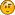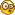###Author Topic: Object3D generating issue  (Read 1147 times)

####AeroShark333

• long
•• Posts: 209##### Object3D generating issue
« on: July 23, 2018, 06:05:48 pm »
Hello,

I've been trying to create some code to generate a geosphere/icosphere, I got somewhere but the results aren't exactly perfect.
Issue 1: UV mapping still does not seem perfect (especially around the top and bottom, where texCoord.y is around 0.0 or 1.0) but around the middle (0.5) all seems fine
Issue 2: I seem to get black polygons on some devices (but I don't get these black polygons on an emulator..?)

The code till now (it's not really optimized yet since I ported most of the code from some C# code: http://blog.andreaskahler.com/2009/06/creating-icosphere-mesh-in-code.html):
Code: [Select]
`private class TriangleIndices { public int v1; public int v2; public int v3; public TriangleIndices(int v1, int v2, int v3) { this.v1 = v1; this.v2 = v2; this.v3 = v3; } } private ArrayList<SimpleVector> vertexData; private ArrayList<Integer> vertexDataIndices; private int index; private Map<Long, Integer> middlePointIndexCache; // add vertex to mesh, fix position to be on unit sphere, return index private int addVertex(SimpleVector p) {// 0.5 is a scale factor here... double length = Math.sqrt(p.x * p.x + p.y * p.y + p.z * p.z) / 0.5; vertexData.add(new SimpleVector(p.x / length, p.y / length, p.z / length)); return index++; } // return index of point in the middle of p1 and p2 private int getMiddlePoint(int p1, int p2) { // first check if we have it already boolean firstIsSmaller = p1 < p2; long smallerIndex = firstIsSmaller ? p1 : p2; long greaterIndex = firstIsSmaller ? p2 : p1; long key = (smallerIndex << 32) + greaterIndex; if (middlePointIndexCache.containsKey(key)) { int ret = this.middlePointIndexCache.get(key); return ret; } // not in cache, calculate it SimpleVector point1 = this.vertexData.get(p1); SimpleVector point2 = this.vertexData.get(p2); SimpleVector middle = new SimpleVector((point1.x + point2.x) / 2.0, (point1.y + point2.y) / 2.0, (point1.z + point2.z) / 2.0); // add vertex makes sure point is on unit sphere int i = addVertex(middle); // store it, return index this.middlePointIndexCache.put(key, i); return i; } public Object3D create(int recursionLevel) { this.vertexData = new ArrayList<SimpleVector>(); this.vertexDataIndices = new ArrayList<Integer>(); this.middlePointIndexCache = new HashMap<Long, Integer>(); this.index = 0; // create 12 vertices of a icosahedron float t = (float) ((1.0f + Math.sqrt(5.0f)) / 2.0f); addVertex(new SimpleVector(-1, t, 0)); addVertex(new SimpleVector(1, t, 0)); addVertex(new SimpleVector(-1, -t, 0)); addVertex(new SimpleVector(1, -t, 0)); addVertex(new SimpleVector(0, -1, t)); addVertex(new SimpleVector(0, 1, t)); addVertex(new SimpleVector(0, -1, -t)); addVertex(new SimpleVector(0, 1, -t)); addVertex(new SimpleVector(t, 0, -1)); addVertex(new SimpleVector(t, 0, 1)); addVertex(new SimpleVector(-t, 0, -1)); addVertex(new SimpleVector(-t, 0, 1)); // create 20 triangles of the icosahedron List<TriangleIndices> faces = new ArrayList<TriangleIndices>(); // 5 faces around point 0 faces.add(new TriangleIndices(0, 11, 5)); faces.add(new TriangleIndices(0, 5, 1)); faces.add(new TriangleIndices(0, 1, 7)); faces.add(new TriangleIndices(0, 7, 10)); faces.add(new TriangleIndices(0, 10, 11)); // 5 adjacent faces faces.add(new TriangleIndices(1, 5, 9)); faces.add(new TriangleIndices(5, 11, 4)); faces.add(new TriangleIndices(11, 10, 2)); faces.add(new TriangleIndices(10, 7, 6)); faces.add(new TriangleIndices(7, 1, 8)); // 5 faces around point 3 faces.add(new TriangleIndices(3, 9, 4)); faces.add(new TriangleIndices(3, 4, 2)); faces.add(new TriangleIndices(3, 2, 6)); faces.add(new TriangleIndices(3, 6, 8)); faces.add(new TriangleIndices(3, 8, 9)); // 5 adjacent faces faces.add(new TriangleIndices(4, 9, 5)); faces.add(new TriangleIndices(2, 4, 11)); faces.add(new TriangleIndices(6, 2, 10)); faces.add(new TriangleIndices(8, 6, 7)); faces.add(new TriangleIndices(9, 8, 1)); // refine triangles for (int i = 0; i < recursionLevel; i++) { List<TriangleIndices> faces2 = new ArrayList<TriangleIndices>(); for (TriangleIndices tri : faces) { // replace triangle by 4 triangles int a = getMiddlePoint(tri.v1, tri.v2); int b = getMiddlePoint(tri.v2, tri.v3); int c = getMiddlePoint(tri.v3, tri.v1); faces2.add(new TriangleIndices(tri.v1, a, c)); faces2.add(new TriangleIndices(tri.v2, b, a)); faces2.add(new TriangleIndices(tri.v3, c, b)); faces2.add(new TriangleIndices(a, b, c)); } faces = faces2; } // done, now add triangles to mesh for (TriangleIndices tri : faces) { this.vertexDataIndices.add(tri.v1); this.vertexDataIndices.add(tri.v2); this.vertexDataIndices.add(tri.v3); } Object3D obj = new Object3D(vertexDataIndices.size() / 3); for (int c = 0; c < (vertexDataIndices.size() / 3); c++) { final SimpleVector aa = vertexData .get(vertexDataIndices.get(c * 3)); final SimpleVector aaP = cartesianToPolar(aa, true); final SimpleVector bb = vertexData.get(vertexDataIndices .get(c * 3 + 1)); final SimpleVector bbP = cartesianToPolar(bb, true); final SimpleVector cc = vertexData.get(vertexDataIndices .get(c * 3 + 2)); final SimpleVector ccP = cartesianToPolar(cc, true); // texture corrections... if (Math.abs(aaP.z - bbP.z) > 0.5f) { if(aaP.z>bbP.z){ aaP.z-=1.0f; }else{ bbP.z-=1.0f; } } if (Math.abs(aaP.z - ccP.z) > 0.5f) { if(aaP.z>ccP.z){ aaP.z-=1.0f; }else{ ccP.z-=1.0f; } } if (Math.abs(bbP.z - ccP.z) > 0.5f) { if(ccP.z>bbP.z){ ccP.z-=1.0f; }else{ bbP.z-=1.0f; } } obj.addTriangle(aa, aaP.z, aaP.y, bb, bbP.z, bbP.y, cc, ccP.z, ccP.y); } obj.getMesh().getTriangleCount(); return obj; } public static SimpleVector cartesianToPolar(SimpleVector cartesian, boolean normalize) { if (normalize) { return new SimpleVector( cartesian.length(), 1.0 - (Math.acos(cartesian.y / cartesian.length()) / Math.PI), 1.0 - (Math.atan2(cartesian.x, cartesian.z) + Math.PI / 2.0) / (Math.PI * 2.0) ); } return new SimpleVector(cartesian.length(), Math.acos(cartesian.z / cartesian.length()), Math.atan2(cartesian.y, cartesian.x)); }`
Any idea what I'm doing wrong here?

####AeroShark333

• long
•• Posts: 209##### Re: Object3D generating issue
« Reply #1 on: July 27, 2018, 02:19:29 am »
Hmmm?####EgonOlsen

••• Posts: 12035##### Re: Object3D generating issue
« Reply #2 on: July 27, 2018, 10:15:58 am »
No idea about the UV-mapping...about the black polygons? Are you using shaders? If so, that's most likely the problem. Different GPUs show different behaviours in some edge cases and in some cases, it might result in just a black rendering. So...are you using shaders and which devices are affected?

####AeroShark333

• long
•• Posts: 209##### Re: Object3D generating issue
« Reply #3 on: July 29, 2018, 03:39:06 pm »
No idea about the UV-mapping...about the black polygons? Are you using shaders? If so, that's most likely the problem. Different GPUs show different behaviours in some edge cases and in some cases, it might result in just a black rendering. So...are you using shaders and which devices are affected?
Yeah, I'm using shaders (I kind of found out that that's the issue most likely)

I was kind of surprised because it happens everywhere on the Object3D
As can be seen here: https://www.dropbox.com/s/yuxik4jih3dq107/Screenshot_Art_of_Earthify_20180729-151321.png?dl=0

When I use another (simple) fragment shader, the problem does not happen
Code: [Select]
`void main(){gl_FragColor = vec4(vec3(texCoord.y)*texCoord.x,1.0);}`But it kind of does reveal why the UV mapping isn't perfect, it's just missing triangles on the border which the original code doesn't provide...
Screenshot 1: https://www.dropbox.com/s/0ko53cmwjsmakfh/Screenshot_Interactieve_achtergrondkiezer_20180729-150640.png?dl=0
Screenshot 3: https://www.dropbox.com/s/o8vv334dm0yiua7/Screenshot_Interactieve_achtergrondkiezer_20180729-150711.png?dl=0

So yeah, around the middle all seems fine but around the top and bottom there is a zigzagging effect around the top and bottom (texCoord.y variable)...
Proper solution would be to add triangles on the center of that zigzagging line I guess
Another 'solution' would be to increase the recursionLevel (see original code in first post) so much that the zigzagging effect becomes smaller...
Final 'solution' would be to 'correct' the texCoord var at that point
I'm not sure if it can just be corrected by changing the way I assign UV coordinates to the triangles in the Java code.

My device:
« Last Edit: July 29, 2018, 03:42:26 pm by AeroShark333 »

####EgonOlsen

••• Posts: 12035##### Re: Object3D generating issue
« Reply #4 on: July 30, 2018, 09:50:29 am »
Some shader compilers for some chips can't handle some code...which can result in everything going black. Here's an older but (not comprehensive) list of what can go wrong: http://www.jpct.net/wiki/index.php?title=GPU_guide

To track that down, I think you need a device that shows the problem and start to remove stuff from the shader or move things around and see what happens.

####AeroShark333

• long
•• Posts: 209##### Re: Object3D generating issue
« Reply #5 on: February 13, 2020, 02:21:41 pm »
I've noticed now that it perhaps is defects in the generated model, and I wonder if Object3D.addTriangle works by adding triangles that are not adjacent to other triangles (so basically floating triangles)
Also, does it matter in what order you define the 3 vertices anti clockwise.
Is there a difference between v3 -> v2 -> v1 and v1 -> v3 -> v2?

Because I seem to get lines in my wireframe model which I don't think I have defined
« Last Edit: February 13, 2020, 02:28:51 pm by AeroShark333 »

####EgonOlsen

••• Posts: 12035##### Re: Object3D generating issue
« Reply #6 on: February 14, 2020, 09:14:53 am »
addTriangle tries to find matching points in the model to reuse those, but if there are none and the triangle is basically "floating", then it will just create three new points. Also order doesn't matter. I don't see how it can be responsible for stray lines.

####AeroShark333

• long
•• Posts: 209##### Re: Object3D generating issue
« Reply #7 on: February 14, 2020, 02:23:30 pm »
I don't really know either why this happens...
When I draw the wireframe model, I can see some 'defects' inside the model that I believe shouldn't actually be there.
When rendered normally (no wireframe), the model shows up normally on the emulator but on the phone it will have missing triangles (holes basically).
I fixed this problem for now by using Object3D#forceGeometryIndices() which doesn't give any problem with the rendering of the model. (but the wireframe model is actually still messed up)

EDIT: https://www.dropbox.com/s/kxyeu61vz9s8nht/bug.png?dl=0
Here's the messy model I was talking about (radius = 0.5f). It's not just these two lines, there's much more (also shorter ones)...
Whenever I check for the distances between all vertices before calling Object3D#addTriangle(), it does not show me any signs of vertices having distances to other vertices that are about the length of these defect lines. In fact the min and max lengths between vertices is: min:0.034591455 max:0.041302014
While I'd estimate that these defect lines are bigger that the radius of 0.5f... Weird..?« Last Edit: February 14, 2020, 03:03:50 pm by AeroShark333 »

####EgonOlsen

••• Posts: 12035##### Re: Object3D generating issue
« Reply #8 on: February 15, 2020, 04:46:17 pm »
Well, regarding wireframe on jPCT-AE, that how it is, I'm afraid. See, for example, here: http://www.jpct.net/forum2/index.php?topic=3562.0

####AeroShark333

• long
•• Posts: 209##### Re: Object3D generating issue
« Reply #9 on: February 15, 2020, 06:53:25 pm »
And regarding why the normal rendering doesn't show 'defects' when Object3D#forceGeometryIndices() is enabled?

####EgonOlsen

••